# 1664 and Level 2

### Today’s Puzzle:

Write the numbers 1 to 12 in both the first column and the top row so that those numbers and the given clues function like a multiplication table.### Factor Cake for 1664:

We can make a factor cake for 1664 by doing some successive divisions. Divide 1664 by 2, divide that answer by 2, and so forth until you make a factor cake that looks like this:### Factors of 1664:

• 1664 is a composite number.
• Prime factorization: 1664 = 2 × 2 × 2 × 2 × 2 × 2 × 2 × 13, which can be written 1664 = 2⁷ × 13.
• 1664 has at least one exponent greater than 1 in its prime factorization so √1664 can be simplified. Taking the factor pair from the factor pair table below with the largest square number factor, we get √1664 = (√64)(√26) = 8√26.
• The exponents in the prime factorization are 7 and 1. Adding one to each exponent and multiplying we get (7 + 1)(1 + 1) = 8 × 2 = 16. Therefore 1664 has exactly 16 factors.
• The factors of 1664 are outlined with their factor pair partners in the graphic below.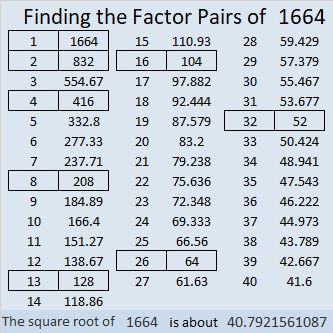### More About the Number 1664:

1664 is the sum of two squares:
40² + 8² = 1664.
That happened because it has a prime factor that leaves a remainder of 1 when divided by 4 AND all of its other prime factors are powers of 2 or perfect squares:

But that’s not all that cool about 1664. What patterns do you notice below?
2(24² + 16²) = 1664,
4(20² + 4²) = 1664,
8(12² + 8²) = 1664,
16(10² + 2²) = 1664,
32(6² + 4²) = 1664,
64(5² + 1²) = 1664, and
128(3² + 2²) = 1664.

1664 is the hypotenuse of a Pythagorean triple:
640-1536-1664, calculated from 2(40)(8), 40² – 8², 40² + 8².
That triple is also (5-12-13) times 128.

# 1512 Bigger Bites of Cake

### Using the Cake Method:

I like using the cake method to find the prime factorization of a number. I also use it to find square roots.

If you know the multiplication table well, dividing by any number from 2 to 9 is not difficult.

It is easy to check to see if a number is divisible by 4 or by 9. And it is actually easier to divide by 4 once than it is to divide by 2 twice or to divide by 9 once than it is to divide by 3 twice. That way we get to take bigger bites of cake!

I know that 1512 is divisible by 4 because the number formed from the last two digits in order, 12, is divisible by 4.

I also know that 1512 is divisible by 9 because 1 + 5 + 1 + 2 = 9.

Thus, I’ll begin by dividing first by 4 and then the result by 9 as illustrated below: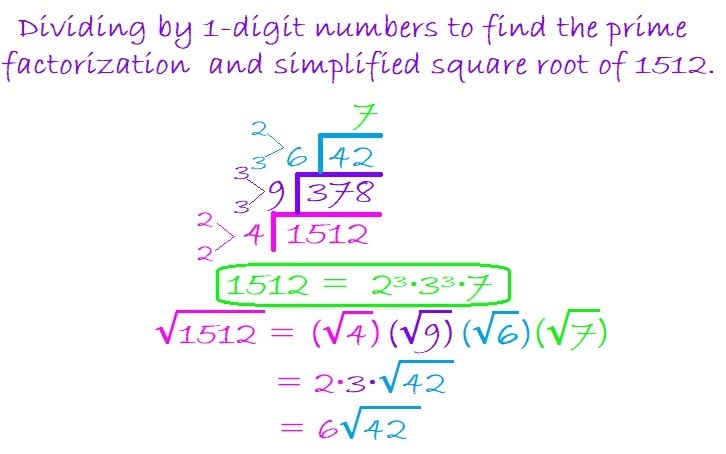When my new divisor becomes 42, if I didn’t remember where it appears in the multiplication table, I would still be fine. I know that 42 is divisible by 2 because it is even and 3 because 4 + 2 = 6, a number divisible by 3.

Thus I can divide 42 by 6 because 2 × 3 = 6, and it is far easier to divide 42 by 6 than it is to divide it first by 2 and then by 3.

To take the square root of 1512, I simply take the square root of the numbers on the outside of the cake. 4 and 9 are perfect squares so I use their square roots. Neither 6 nor 7 has any perfect squares, so I just multiply them together to get 42.

Dividing 1512 this way allowed me to make just a three-layer cake instead of a 6-layer cake to find its prime factorization and its square root!

Some people prefer to do yard work more than having cake, so here is one of the MANY possible factor trees for 1512. Make sure you pick up all seven of the leaves with prime numbers wherever they are.### Factors of 1512:

• 1512 is a composite number.
• Prime factorization: 1512 = 2 × 2 × 2 × 3 × 3 × 3 × 7, which can be written 1512 = 2³ × 3³ × 7.
• 1512 has at least one exponent greater than 1 in its prime factorization so √1512 can be simplified. Taking the factor pair from the factor pair table below with the largest square number factor, we get √1512 = (√36)(√42) = 6√42.
• The exponents in the prime factorization are 2, 1, and 2. Adding one to each exponent and multiplying we get (3 + 1)(3 + 1)(1 + 1) = 4 × 4 × 2 = 32. Therefore 1512 has exactly 32 factors.
• The factors of 1512 are outlined with their factor pair partners in the graphic below.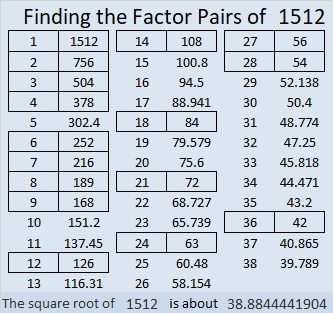# 1386 What You Need to Know About the Multiplication Game

I recently wrote about Hooda Math’s Multiplication Game. There’s a couple of things I didn’t tell you in that post.

First of all, you don’t have to use a computer to play the game. (However, using one the first time you play will help you understand how to play). You can print a game board to play. I’ve created a game board below that you could use. Each player can use different items such as beads, pennies, nickels, and dimes as markers to mark the factors used and to claim the resulting products on the game board.The second thing you should know is that getting four squares in a row, horizontally, vertically or diagonally is NOT equally likely every place on the board. If one particular number is all you need to get a win, you are less likely to get that number if it only has one factor (like the numbers marked in yellow have). As far as this game is concerned, the products have the number of factors that I’ve indicated, even though in reality most of them have more than that.

You can’t win unless your opponent gives you one of the factors you need to claim that winning space. If 4 of the 9 possible factors will get it for you, the odds are much better your opponent will give you what you need than if only 1 of the 9 possible factors will do it.

If you know which numbers have four possible factors, you may have an advantage over someone who thinks this game is really just a variation of tic-tac-toe. Of course, those products with four factors could also make you more likely to get blocked as well! And if you use my colorful game board, your opponent will know just as much as you do about how many ways they can get each square.

### 1386 Factor Cake:

Since the biggest prime factor of 1386 is 11, it makes an especially festive factor cake!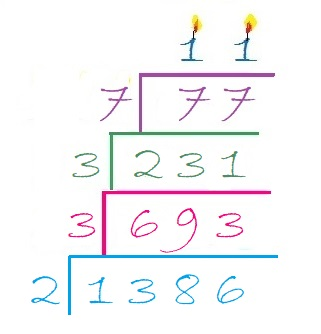### Factors of 1386:

Now I’ll share some information about the number 1386:

• 1386 is a composite number.
• Prime factorization: 1386 = 2 × 3 × 3 × 7 × 11, which can be written 1386 = 2 × 3² × 7 × 11
• 1386 has at least one exponent greater than 1 in its prime factorization so √1386 can be simplified. Taking the factor pair from the factor pair table below with the largest square number factor, we get √1386 = (√9)(√154) = 3√154
• The exponents in the prime factorization are 1, 2, 1, and 1. Adding one to each exponent and multiplying we get (1 + 1)(2 + 1)(1 + 1)(1 + 1) = 2 × 3 × 2 × 2 = 24. Therefore 1386 has exactly 24 factors.
• The factors of 1386 are outlined with their factor pair partners in the graphic below.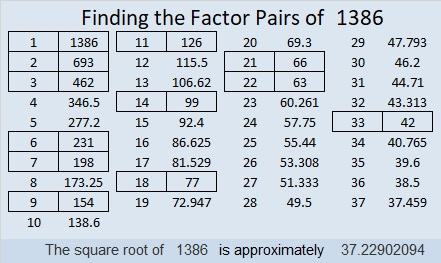### Sum-Difference Puzzle:

1386 has twelve factor pairs. One of the factor pairs adds up to 85, and a different one subtracts to 85. If you can identify those factor pairs, then you can solve this puzzle!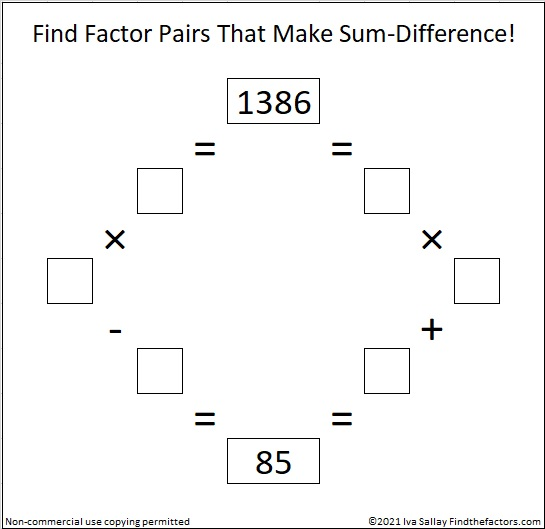### One More Fact about the Number 1386:

OEIS.org also noted that 1 + 3⁴ + 8 + 6⁴ = 1386.

# 1232 Factor Cake

1232 is divisible by 2 because it’s even.
It’s divisible by 4 because 32 is divisible by 4
It’s divisible by 8 because 232 is divisible by 8.
It also happens to be divisible by 16 and by 7.
It’s divisible by 11 because 1 – 2 + 3 – 2 = 0

1232 makes a delicious-looking factor cake:From the factor cake, we see that 2 · 2 · 2 · 2 · 7 · 11 = 1232.

Here’s more about the number 1232:

• 1232 is a composite number.
• Prime factorization: 1232 = 2 × 2 × 2 × 2 × 7 × 11, which can be written 1232 = 2⁴ × 7 × 11
• The exponents in the prime factorization are 4, 1 and 1. Adding one to each and multiplying we get (4 + 1)(1 + 1)(1 + 1) = 5 × 2 × 2 = 20. Therefore 1232 has exactly 20 factors.
• Factors of 1232: 1, 2, 4, 7, 8, 11, 14, 16, 22, 28, 44, 56, 77, 88, 112, 154, 176, 308, 616, 1232
• Factor pairs: 1232 = 1 × 1232, 2 × 616, 4 × 308, 7 × 176, 8 × 154, 11 × 112, 14 × 88, 16 × 77, 22 × 56, or 28 × 44
• Taking the factor pair with the largest square number factor, we get √1232 = (√16)(√77) = 4√77 ≈ 35.09986The odd prime factors of 1232 are 7 and 11.
OEIS.org informs us that (7 × 8 × 9 × 10 × 11) / (7 + 8 + 9 + 10 + 11) = 1232

# Reasons to Celebrate 1155

1155 is the product of the four smallest odd prime numbers. That alone is a reason to celebrate. Here, I’ve made a factor cake with 1155 for you to enjoy!make science GIFs like this at MakeaGif

Besides 1155 being the smallest number divisible by the first 4 odd prime numbers, what else can I say about it?

• 1155 is a composite number.
• Prime factorization: 1155 = 3 × 5 × 7 × 11
• The exponents in the prime factorization are 1, 1, 1, and 1. Adding one to each and multiplying we get (1 + 1)(1 + 1)(1 + 1)(1 + 1) = 2 × 2 × 2 × 2 = 16. Therefore 1155 has exactly 16 factors.
• Factors of 1155: 1, 3, 5, 7, 11, 15, 21, 33, 35, 55, 77, 105, 165, 231, 385, 1155
• Factor pairs: 1155 = 1 × 1155, 3 × 385, 5 × 231, 7 × 165, 11 × 105, 15 × 77, 21 × 55, or 33 × 35
• 1155 has no square factors that allow its square root to be simplified. √1155 ≈ 33.98529Since it’s number of prime factors is a power of 2, it can make some very well-balanced and attractive factor trees:1155 is the hypotenuse of a Pythagorean triple:
693-924-1155 which is (3-4-5) times 231

1155 is one number away from the next perfect square, AND it is the difference of two squares EIGHT different ways.
34² – 1² = 1155
38² – 17² = 1155
46² – 31² = 1155
58² – 47² = 1155
86² – 79² = 1155
118² – 113² = 1155
194² – 191² = 1155
578² – 577² = 1155

Those eight ways correspond to its eight factor pairs. Notice that both of the numbers in all of its factor pairs are odd numbers.

I like the way 1155 looks in some other bases:
It’s 3A3 in BASE 18 (A is 10 base 10) because 3(18²) + 10(18) + 3(1) = 1155
XX in BASE 34 (X is 33 base 10) because 33(34) + 33(1) = 33(35) = 1155
X0 in BASE 35 because 33(35) = 1155

XX X0 . . . Hugs and Kisses. You gotta love that!

# 1144 Let’s Make a Factor Cake!

Occasionally, I’ll put a factor cake on this blog, but until today, I haven’t really shown you how to MAKE a factor cake. I made this simple gif to show you how to make a factor cake from start to finish. You can watch it over and over again if you like. Just repeatedly divide by the smallest prime number divisor.make science GIFs like this at MakeaGif

Here’s more about the number 1144:

• 1144 is a composite number.
• Prime factorization: 1144 = 2 × 2 × 2 × 11 × 13, which can be written 1144 = 2³ × 11 × 13
• The exponents in the prime factorization are 3, 1, and 1. Adding one to each and multiplying we get (3 + 1)(1 + 1)(1 + 1) = 4 × 2 × 2 = 16. Therefore 1144 has exactly 16 factors.
• Factors of 1144: 1, 2, 4, 8, 11, 13, 22, 26, 44, 52, 88, 104, 143, 286, 572, 1144
• Factor pairs: 1144 = 1 × 1144, 2 × 572, 4 × 286, 8 × 143, 11 × 104, 13 × 88, 22 × 52, or 26 × 44
• Taking the factor pair with the largest square number factor, we get √1144 = (√4)(√286) = 2√286 ≈ 33.82307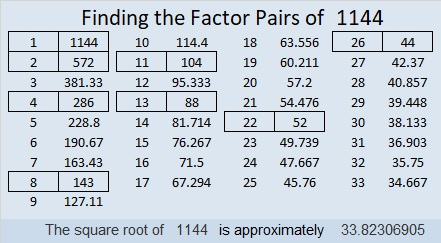1144 is obviously divisible by 11, but it isn’t so obvious that 1144 is the sum of the twenty-two prime numbers from 11 to 101.

1144 is the hypotenuse of a Pythagorean triple:
440-1056-1144 which is (5-12-13) times 88

1144 is palindrome 3223 in BASE 7 because 3(7³) + 2(7²) + 2(7) +3(1) = 1144

# 1125 May I Interest You In a Little Cake?

1 + 1 + 2 + 5 = 9, so 1125 can be evenly divided by both 3 and 9.
1125 can also be evenly divided by 5 because the last digit can be divided by 5.
1125 can be evenly divided by 25 because the last two digits can be divided by 25.
1125 can be evenly divided by 125 because the last three digits can be divided by 125.

May I interest you in a little cake? Although I’ve put many factor trees on this website, I actually prefer making factor cakes. I like them because all the prime factors can easily be found IN ORDER on the outside of the cake. The more layers the factor cake has, the more I like it. Here is THE factor cake for 1125: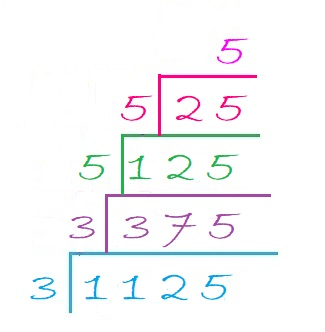As you can see, the prime factorization of 1125 is 3 × 3 × 5 × 5 × 5. All of its prime factors are written nicely in order. None of them are hiding anyplace like they might in a factor tree.

Here are some facts about the number 1125:

• 1125 is a composite number.
• Prime factorization: 1125 = 3 × 3 × 5 × 5 × 5, which can be written 1125 = 3² × 5³
• The exponents in the prime factorization are 2 and 3. Adding one to each and multiplying we get (2 + 1)(3 + 1) = 3 × 4 = 12. Therefore 1125 has exactly 12 factors.
• Factors of 1125: 1, 3, 5, 9, 15, 25, 45, 75, 125, 225, 375, 1125
• Factor pairs: 1125 = 1 × 1125, 3 × 375, 5 × 225, 9 × 125, 15 × 75, or 25 × 45
• Taking the factor pair with the largest square number factor, we get √1125 = (√225)(√5) = 15√5 ≈ 33.54102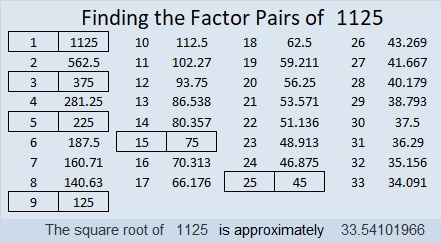1125 is the hypotenuse of three Pythagorean triples:
675-900-1125 which is (3-4-5) times 225,
315-1080-1125 which is (7-24-25) times 45, and
396-1053-1125 which is 9 times (44-117-125)

1125 looks interesting to me when it is written in these bases:
It’s 5A5 in BASE 14 (A is 10 base 10) because 5(14²) + 10(14) + 5(1) = 1125
500 in BASE 15 because 5(15²) = 1125, and
it’s 3F3 in BASE 17 (F is 15 base 10) because 3(17²) + 15(17) + 3(1) = 1125

# 1078 and Level 6

This Level 6 puzzle might be just a little bit easier than usual, so if you’ve never done this level before, be sure to try this one! You can succeed if you stick with it!Print the puzzles or type the solution in this excel file: 10-factors-1073-1079

Here are a few facts about the number 1078:

• 1078 is a composite number.
• Prime factorization: 1078 = 2 × 7 × 7 × 11, which can be written 1078 = 2 × 7² × 11
• The exponents in the prime factorization are 1, 2, and 1. Adding one to each and multiplying we get (1 + 1)(2 + 1)(1 + 1) = 2 × 3 × 2 = 12. Therefore 1078 has exactly 12 factors.
• Factors of 1078: 1, 2, 7, 11, 14, 22, 49, 77, 98, 154, 539, 1078
• Factor pairs: 1078 = 1 × 1078, 2 × 539, 7 × 154, 11 × 98, 14 × 77, or 22 × 49,
• Taking the factor pair with the largest square number factor, we get √1078 = (√49)(√22) = 7√22 ≈ 32.83291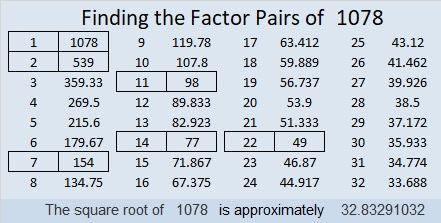1 – 0 + 7 – 8 = 0 so 1078 is divisible by 11.

Since 11 is its largest prime factor we can make a lovely factor cake for 1078:1078 is palindrome 4554 in BASE 6 because 4(6³) + 5(6²) + 5(6) + 4(1) = 1078

# 808 Happy Birthday, Justin!

Happy birthday to my good friend, Justin! He seems to always remember the birthdays of everyone he knows, so this is how I am remembering his special day today. Justin is highly intelligent, thoughtful, and very friendly. I am confident he can solve this Level 6 puzzle that looks a little like a birthday cake.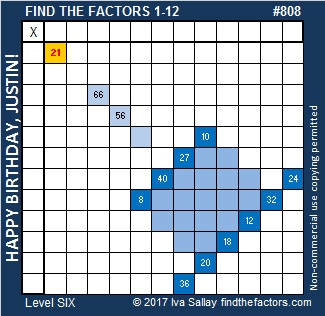Print the puzzles or type the solution on this excel file: 10-factors 807-814

This is my 808th post so I thought I would also make a factor cake for the number 808. It’s prime factor, 101, is at the top of the cake. Justin, I hope you live to be 101!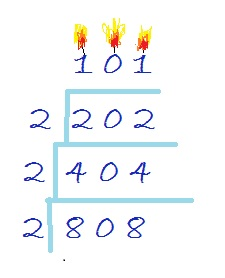808 is a palindrome. That means it looks the same forwards and backwards. It is also a strobogrammatic number. That means it looks the same right side up or upside down.

ALL of the factors of 808 are also palindromes, and four of them are strobogrammatic numbers, too. Can you figure out which ones are both?

• 808 is a composite number.
• Prime factorization: 808 = 2 x 2 x 2 x 101, which can be written 808 = (2^3) x 101
• The exponents in the prime factorization are 3 and 1. Adding one to each and multiplying we get (3 + 1)(1 + 1) = 4 x 2 = 8. Therefore 808 has exactly 8 factors.
• Factors of 808: 1, 2, 4, 8, 101, 202, 404, 808
• Factor pairs: 808 = 1 x 808, 2 x 404, 4 x 202, or 8 x 101
• Taking the factor pair with the largest square number factor, we get √808 = (√4)(√202) = 2√202 ≈ 28.425340807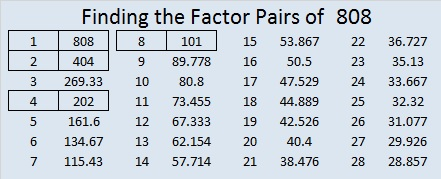Here are the factors that make puzzle #808 act like a multiplication table. It is followed by a table of logical steps to arrive at that solution.